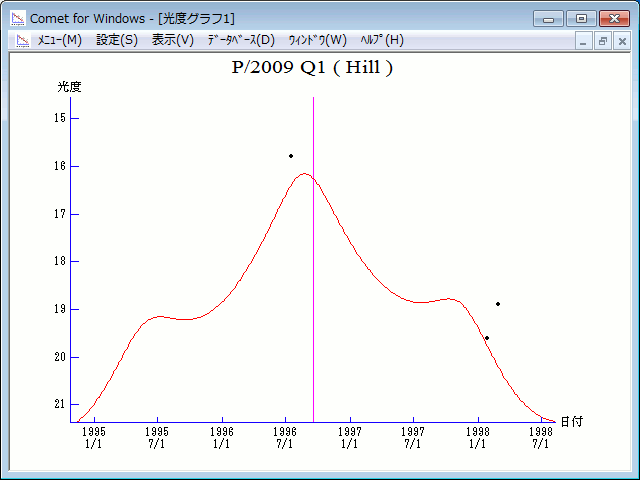# \$B%R%kWB@1(B

420P/Hill (1996)###\$B50F;MWAG(B

```                    Epoch = 1996 Oct.  4.0 TT
T = 1996 Sept.16.29547 TT        Peri. = 159.16042
e = 0.4968608                    Node  = 174.31326 2000.0
q = 2.7593400 AU                 Incl. =  14.45157
a =  5.4842471 AU   n = 0.07674119   P =  12.84 years
```

###\$B8wEYJQ2=(B

```        m1 = 10.5 + 5 log\$B&\$(B + 10.0 log r
```##### \$B50F;MWAG\$O(BCBET 4943\$B\$K7G:\\$5\$l\$?\$b\$N\$G\$9!#(B \$B8wEY%0%i%U\$O(BComet for Windows\$B\$G:n@.\$7\$?\$b\$N\$G\$9!#(B